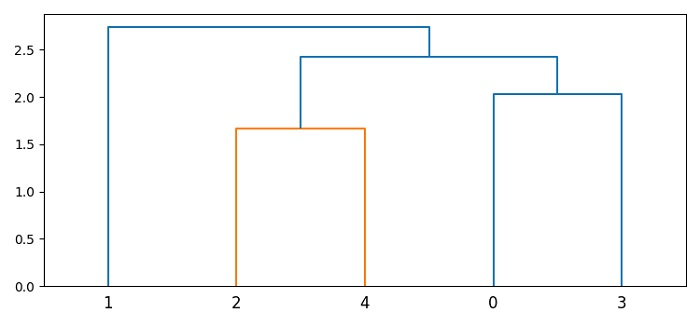# How to adjust the branch lengths of a dendrogram in Matplotlib?

To adjust the branch length of a dendrogram in Matplotlib, we can take the following steps −

• Set the figure size and adjust the padding between and around the subplots.

• Draw random samples (a and b) from a multivariate normal distribution.

• Join a sequence of arrays along an existing axis, using concatenate() method.

• Perform hierarchical/agglomerative clustering.

• Create a new figure or activate an existing figure using figure() method.

• Add an axes to the figure as part of a subplot arrangement.

• Plot the hierarchical clustering as a dendrogram using dendrogram() method.

• To display the figure, use show() method.

## Example

from matplotlib import pyplot as plt
import numpy as np
plt.rcParams["figure.figsize"] = [7.50, 3.50]
plt.rcParams["figure.autolayout"] = True
a = np.random.multivariate_normal([0, 10], [[3, 1], [1, 4]], size=[2, ])
b = np.random.multivariate_normal([0, 10], [[3, 1], [1, 4]], size=[3, ])
X = np.concatenate((a, b), )
fig = plt.figure()
dendrogram(Z, ax=ax)
plt.show()

## OutputUpdated on: 03-Jun-2021

683 Views